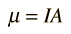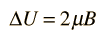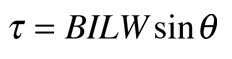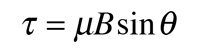# Magnetic Dipole Moment

From the expression for the torque on a current loop, the characteristics of the current loop are summarized in its magnetic moment.

The magnetic moment can be considered to be a vector quantity with direction perpendicular to the current loop in the right-hand-rule direction. The torque is given byAs seen in the geometry of a current loop, this torque tends to line up the magnetic moment with the magnetic field B, so this represents its lowest energy configuration. The potential energy associated with the magnetic moment isso that the difference in energy between aligned and anti-aligned isThese relationships for a finite current loop extend to the magnetic dipoles of electron orbits and to the intrinsic magnetic moment associated with electron spin. Also important are nuclear magnetic moments.

Index

Magnetic force applications

Magnetic field concepts

 HyperPhysics***** Electricity and Magnetism R Nave
Go Back

# Torque on a Current Loop

The torque on a current-carrying coil, as in a DC motor, can be related to the characteristics of the coil by the "magnetic moment" or "magnetic dipole moment". The torque exerted by the magnetic force (including both sides of the coil) is given byThe coil characteristics can be grouped ascalled the magnetic moment of the loop, and the torque written asThe direction of the magnetic moment is perpendicular to the current loop in the right-hand-rule direction, the direction of the normal to the loop in the illustration. Considering torque as a vector quantity, this can be written as the vector productSince this torque acts perpendicular to the magnetic moment, then it can cause the magnetic moment to precess around the magnetic field at a characteristic frequency called the Larmor frequency.

If you exerted the necessary torque to overcome the magnetic torque and rotate the loop from angle zero to 180 degrees, you would do an amount of rotational work given by the integralThe position where the magnetic moment is opposite to the magnetic field is said to have a higher magnetic potential energy.

Index

Magnetic force

Magnetic force applications

 HyperPhysics***** Electricity and Magnetism R Nave
Go Back